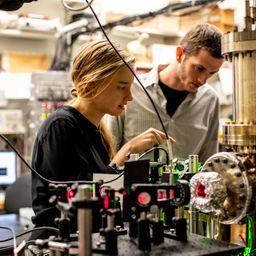## no answer available

Quantum Physics

Atomic Physics

### Discussion

You must be signed in to discuss.
##### Top Physics 103 Educators
LB##### Marshall S.

University of Washington##### Jared E.

University of Winnipeg

### Video Transcript

Okay, so we know that all left an electron bowling ball have a particular amount of kinetic energy. And when I got the dobro oily way blanks, um, for each of them. So let's go ahead and get started on that. So basically, we want to use this formula for that abruptly wavelength in general that Lambda is equal to h overpay. Oh, and so, um, let's see. So thick, p, we can really back to kinetic energy. So Connecticut RGs equal toe, um, p squared over to, um oops, what should be an M and so we can solve for peace. We can say it's a JJ over the square root of two, sometimes the kinetic energy. And so for, um, a we want to get the, um it's an electron until we want to use the mass of the electron. And then, uh, for this calculation, so we can say Lambda E is equal to age over the square root of two. And then now this is gonna be massive the electron, and then this is gonna be Katie. So I guess I could write it up here. This is kind of my everything box, all right? The kinetic energy is 4.5 e v. And yeah, Then we run multiplied by the kinetic energy. Of course, we wantto multiply by 1.6 times 10 to the minus 19. Um, to convert it from on. Jules two converted from individuals. Okay, great. So I'm gonna go ahead and plug this into a calculator, So age 6.626 times 10 to the minus 34. We're all divided by the square root of few times my prayer 11 times, 10 to the minus. Their run times 455 times. 1.6 times. 10 to the minus 19. Um, And with that, I got five point. Let's see, 5.8. How many? Six eggs. 036 figs. Um, so I get 5.79 times 10 to the minus 10 meters. That's it. And we want to do the same for bowling ball. So the only thing we're gonna do is now Emma's equal this six point. 00 we're gonna kind of plug it in the same into this formula. And so, um, and then again would just convert the kinetic energy to Jules and soap lugging all that and we get the lambda for the bowling ball. So it's gonna be, um, significantly smaller because the mass of the bowling ball is significantly larger. So that's gonna just be six. And I got 2.25 times 10 to the minus 25. Yeah, great. And so for See, we now, we kind of wanna compare this toe wavelengths of light. So if we have a 4.5 e v photons on, um, we want to get the wavelength for sometimes we want to do. He is equal to agency over Lambda. And then therefore, at Lambda is HC over e cross multiplying our however you wanna talk about the algebra and, um, again, you want to convert this e v to Jules. So let's go back to my calculator and evaluate this. So 6.626 times, 2.8 times 10 Could be eight. Divided by 4.5 come from behind six times seven minus 19. With that, I got 2.76 times 10 to the minus seven, you know, so 276 nanometers groups. This is getting weirder and weirder and great. ThatUniversity of Washington

#### Topics

Quantum Physics

Atomic Physics

##### Top Physics 103 Educators
LB##### Marshall S.

University of Washington##### Jared E.

University of Winnipeg